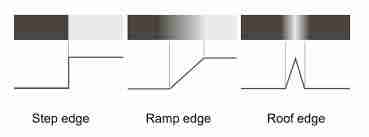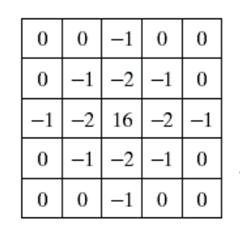# 不使用OpenCV拉普拉斯内建函数的拉普拉斯算子边缘检测。## 代码说明

Mat img = imread(argv, 1);

Mat output = gr.clone();

for (int i = 1; i < height - 1; i++) {

output.at(y，x)=sum；

255+255+255+255-4*0=1020

0+0+0+0-4*255=-1020

#gray picture without laplacian namedWindow("Gray Image");imshow("Gray Image", gr);## 高斯的拉普拉斯式

int sum3 = 0;